×#### Thank you for registering.

One of our academic counsellors will contact you within 1 working day.

Click to Chat

1800-1023-196

+91-120-4616500

CART 0

• 0

MY CART (5)

Use Coupon: CART20 and get 20% off on all online Study Material

ITEM
DETAILS
MRP
DISCOUNT
FINAL PRICE
Total Price: Rs.

There are no items in this cart.
Continue Shopping• Complete JEE Main/Advanced Course and Test Series
• OFFERED PRICE: Rs. 15,900
• View Details

```Chapter 11: Angles Exercise 11.2

Question: 1

Give two examples each of right, acute and obtuse angles from your environment.

Solution:

Two examples of right angle in our environment are:

(i) The angle formed by the two adjacent walls of a room is a right angle.

(ii) The angle formed by the two adjacent edges of a book is a right angle.

Two examples of acute angle in our environment are:

(i) The angle formed between the two adjacent fingers of our hand.

(ii) The angle between the two adjacent sides of the letter Z of English alphabet.

Two examples of obtuse angle in our environment are:

(i) The smaller angle formed by the two adjacent blades of a fan.

(ii) The smaller angle formed by the two sloping sides of a roof of a but is an obtuse angle.

Question: 2

An angle is formed by two adjacent fingers. What kind of angle will it appear?

Solution:

Angle formed by two adjacent fingers will appear as an acute angle.

Question: 3

Shikha is rowing a boat due northeast. In which direction will she be rowing if she turns it through:

(i) a straight angle. (ii) a complete angle.

Solution:

(i) If Shikha turns through a straight angle or 180 degrees, she will be rowing along the south – west direction.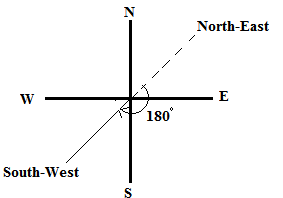(ii) If Shikha turns through a complete angle or 360 degrees, she will be rowing along her original direction, i.e., north – east direction.Question: 4

What is the measure of the angle in degrees between:

(i) North and West?

(ii) North and South?(iii) North and South – East?

Solution:

The measure of the angle between:

(i) North and West is 90 degrees.

(ii) North and South is 180 degrees.

(iii) North and South – East is 135 degrees.

Question: 5

A ship sailing in river Jhelam moves towards east. If it changes to north, through what angle does it turn?

Solution:

If the ship is sailing in east direction and turns to north direction, it turns through an angle of 90 degrees.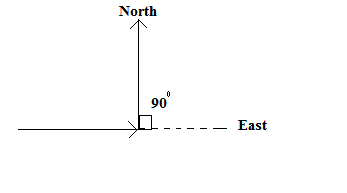Question: 6

You are standing in a class room facing north. In what direction are you facing after making a quarter turn?

Solution:

After making a quarter turn or a turn of 90 degrees, i will be facing east if i turn to my right hand. Similarly, if i turn to my left hand, i will be facing west.

Question: 7

A bicycle wheel makes four and a half turns. Find the number of right angles through which it turns.

Solution:

In one turn, the wheel of a bicycle covers 360°.

If we express 360° in right angles, we get:

360°/90° = 4 right angles.

Thus, in four and a half turns, the wheel will turn by (4 × 4.5) = 18 right angles.

Question: 8

Look at your watch face. Through how many right angles does the minute hand moves between 8 O’ clock and 10:30 O’ clock?

Solution:

The time interval between 8: 00 O’clock and 10 : 30 O’clock is 2.5 hours, i.e., two and a half hours.

In 1 hour, the minute hand turns by a complete angle, i.e., 360° or 360°/90° = 4 right angles.

Thus, in 2.5 hours, the minute hand will turn by 2.5 × 4 = 10 right angles.

Question: 9

If a bicycle wheel has 48 spokes, then find the angle between a pair of adjacent spokes.

Solution:

In a bicycle, the central angle measures 360° and it consists of 48 spokes.

Therefore, angle between any two adjacent spokes = 360/48 = 7.5°.

Question: 10

Classify the following angles as acute, obtuse, straight, right, zero and complete angle:

(i) 118°

(ii) 29°

(iii) 145°

(iv) 165°

(v) 0↑8

(vi) 75°

(vii) 180°

(viii) 89.5°

(ix) 30°

(x) 90°

(xi) 179°

(xii) 360°

(xiii) 90.5°

Solution:

An acute angle measures between 0° and 90°; an obtuse angle measures between 90° and 180°; a straight angle measures 180°; a right angle measures 90°; a zero angle measures 0° and a complete angle measures 360°.

(i) 118° is an obtuse angle.

(ii) 29° is an acute angle.

(iii) 145° is an obtuse angle.

(iv) 165° is an obtuse angle.

(v) 0° is a zero angle.

(vi) 75° is an acute angle.

(vii) 180° is a straight angle.

(viii) 89.5° is an acute angle.

(ix) 30° is an acute angle.

(x) 90° is a right angle.

(xi) 179° is an obtuse angle.

(xii) 360° is a complete angle.

(xiii) 90.5° is an obtuse angle.

Question: 11

Using only a ruler, draw an acute angle, a right angle and an obtuse angle in your notebook and name them.

Solution: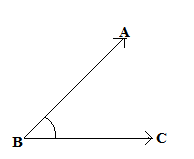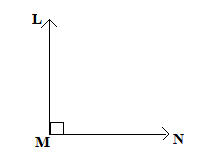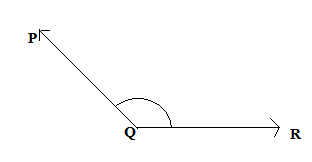Acute angle ∠ABC
Right angle ∠LMN
Obtuse angle ∠PQR

Question: 12

State the kind of angle, in each case, formed between the following directions:

(i) East and West

(ii) East and North

(iii) North and North – East

(iv) North and South – East

Solution:

(i) East and west directions form an angle of 180°, which is a straight angle.

(ii) East and north directions form an angle of 90°, which is a right angle.

(iii) North and north-east directions form an angle of 45°, which is an acute angle.

(iv) North and south-east directions form an angle of 135°, which is an obtuse angle.

Question: 13

State the kind of each of the following angles:

Solution:

(i) Acute angle, as it measures between 0° and 90°.

(ii) Obtuse angle, as it measures between 90° and 180°.

(iii) Straight angle, as it is equal to 180°.

(iv) Right angle, as it is equal to 90°.

(v) Complete angle, as it is equal to 360°.

Objective Type Questions

Mark the correct alternative in each of the following:

Question: 1

The vertex of an angle lies

(a) in its interior (b) in its exterior (c) on the angle (d) inside the angle

Solution:

(c) on the angle.

The vertex of an angle lies on the angle.

Question: 2

The figure formed by two rays with the same initial point is known as

(a) a ray (b) a line (c) an angle (d) a line segment

Solution:

(c) an angle.

An angle is a figure by two rays with the same initial point.

Question: 3

An angle of measure 0° is called

(a) a complete angle (b) a right angle (c) a straight angle (d) none of these

Solution:

(d) none of these.

An angle of measure 0° is called a zero angle.

Question: 4

An angle of measure 90° is called

(a) a complete angle (b) a right angle (c) a straight angle (d) a reflex angle

Solution:

(b) a right angle.

An angle of measure 90° is called a right angle.

Question: 5

An angle of measure 180° is called

(a) a zero angle (b) a right angle (c) a straight angle (d) a reflex angle

Solution:

(c) a straight angle.

An angle of measure 180° is a straight angle.

Question: 6

An angle of measure 360° is called

(a) a zero angle (b) an straight angle (c) a reflex angle (d) a complete angle

Solution:

(d) a complete angle.

An angle of measure 360° is called a complete angle.

Question: 7

An angle of measure 240°is

(a) an acute angle (b) an obtuse angle (c) a straight angle (d) a complete angle

Solution:

None of the given options are correct.

An angle of measure 240°is called a reflex angle.

Question: 8

A reflex angle measures

(a) more than 90° but less than 180° (b) more than 180° but less than 270° (c) more than 180° but less than 360° (d) none of these.

Solution:

(c) more than 180° but less than 360°

A reflex angle is defined as an angle that measures more than 180° but less than 360°.

Question: 9

The number of degrees in 2 right angles is

(a) 90° (b) 180° (c) 270° (d) 360°

Solution:

(b) 180°

Since, 1 right angle = 90°

Therefore, 2 right angles = 90° x 2 = 180°

Question: 10

The number of degrees in 3/2 right angles is

(a) 180° (b) 360° (c) 270° (d) 90°

Solution:

None of the options are correct.

Since, 1 right angle = 90°

Therefore, 3/2 right angles = 3/2 x 90° = 135°

Question: 11

If bicycle wheel has 36 spokes, then the angle between a pair of adjacent spokes is

(a) 10° (b) 15° (c) 20° (d) 12°

Solution:

(a) 10°

The complete angle of bicycle wheel measures is 360°.

Therefore, the angle between two adjacent spokes of the containing 36 spokes = 360/36 = 10°.
```### Course Features

• 728 Video Lectures
• Revision Notes
• Previous Year Papers
• Mind Map
• Study Planner
• NCERT Solutions
• Discussion Forum
• Test paper with Video Solution